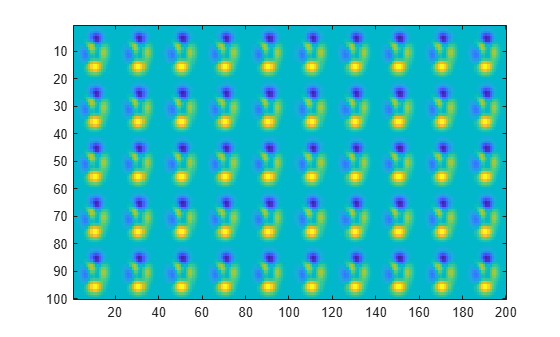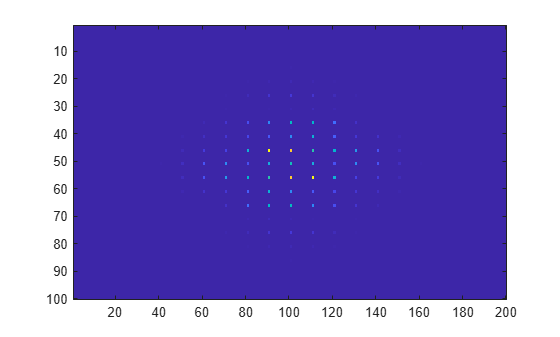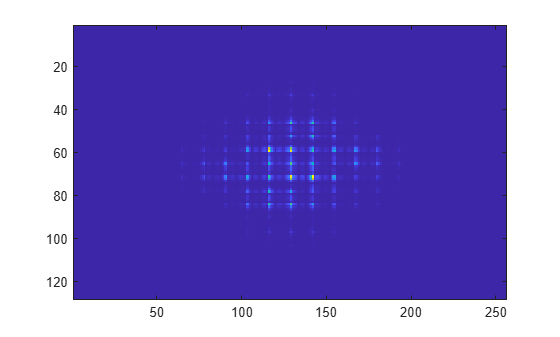# fft2

2-D fast Fourier transform

## Syntax

``Y = fft2(X)``
``Y = fft2(X,m,n)``

## Description

example

````Y = fft2(X)` returns the two-dimensional Fourier transform of a matrix using a fast Fourier transform algorithm, which is equivalent to computing `fft(fft(X).').'`. If `X` is a multidimensional array, then `fft2` takes the 2-D transform of each dimension higher than 2. The output `Y` is the same size as `X`.```

example

````Y = fft2(X,m,n)` truncates `X` or pads `X` with trailing zeros to form an `m`-by-`n` matrix before computing the transform. `Y` is `m`-by-`n`. If `X` is a multidimensional array, then `fft2` shapes the first two dimensions of `X` according to `m` and `n`.```

## Examples

collapse all

The 2-D Fourier transform is useful for processing 2-D signals and other 2-D data such as images.

Create and plot 2-D data with repeated blocks.

```P = peaks(20); X = repmat(P,[5 10]); imagesc(X)```Compute the 2-D Fourier transform of the data. Shift the zero-frequency component to the center of the output, and plot the resulting 100-by-200 matrix, which is the same size as `X`.

```Y = fft2(X); imagesc(abs(fftshift(Y)))```Pad `X` with zeros to compute a 128-by-256 transform.

```Y = fft2(X,2^nextpow2(100),2^nextpow2(200)); imagesc(abs(fftshift(Y)));```## Input Arguments

collapse all

Input array, specified as a matrix or a multidimensional array. If `X` is of type `single`, then `fft2` natively computes in single precision, and `Y` is also of type `single`. Otherwise, `Y` is returned as type `double`.

Data Types: `double` | `single` | `int8` | `int16` | `int32` | `uint8` | `uint16` | `uint32` | `logical`
Complex Number Support: Yes

Number of transform rows, specified as a positive integer scalar.

Data Types: `double` | `single` | `int8` | `int16` | `int32` | `uint8` | `uint16` | `uint32` | `logical`

Number of transform columns, specified as a positive integer scalar.

Data Types: `double` | `single` | `int8` | `int16` | `int32` | `uint8` | `uint16` | `uint32` | `logical`

collapse all

### 2-D Fourier Transform

This formula defines the discrete Fourier transform Y of an m-by-n matrix X:

`${Y}_{p+1,q+1}=\sum _{j=0}^{m-1}\sum _{k=0}^{n-1}{\omega }_{m}^{jp}{\omega }_{n}^{kq}{X}_{j+1,k+1}$`

ωm and ωn are complex roots of unity:

`$\begin{array}{l}{\omega }_{m}={e}^{-2\pi i/m}\\ {\omega }_{n}={e}^{-2\pi i/n}\end{array}$`

i is the imaginary unit. p and j are indices that run from 0 to m–1, and q and k are indices that run from 0 to n–1. This formula shifts the indices for X and Y by 1 to reflect matrix indices in MATLAB®.

## Version History

Introduced before R2006a